Thursday, January 9, 2014

ggplot2: Cheatsheet for Barplots

>> Aggregate data for barplot

In the second of the series, this post will go over barplots in ggplot2. Our data is from mtcars as before.

library(ggplot2)
library(gridExtra)
mtc <- mtcars
# preview data
##                    mpg cyl disp  hp drat    wt  qsec vs am gear carb
## Mazda RX4         21.0   6  160 110 3.90 2.620 16.46  0  1    4    4
## Mazda RX4 Wag     21.0   6  160 110 3.90 2.875 17.02  0  1    4    4
## Datsun 710        22.8   4  108  93 3.85 2.320 18.61  1  1    4    1
## Hornet 4 Drive    21.4   6  258 110 3.08 3.215 19.44  1  0    3    1
## Hornet Sportabout 18.7   8  360 175 3.15 3.440 17.02  0  0    3    2
## Valiant           18.1   6  225 105 2.76 3.460 20.22  1  0    3    1

To introduce the barplot, I show the basic default bargraph that you would get if you indicate an x-variable and use the default geom_bar layer, which is geom_bar(stat=“bin”). You could just write geom_bar() and it would also work. Remember that in ggplot we add layers to make plots, so first we specify the data we want to use and then we specify that we want to plot it as a bar graph (instead of points or lines). The basic plot gives a count of the number in each group of the x-variable (gears).

ggplot(mtc, aes(x = factor(gear))) + geom_bar(stat = "bin")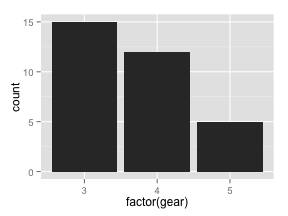>> Aggregate data for barplot

Instead of this, we would like to graphically show the mean weight of the cars by the number of gears. There are a number of ways to make this graph. The first way is that we summarize the data beforehand, and use the summarized data in the ggplot statement. I show two ways to summarize here, with two different results of how the data looks when summarized, using aggregate and tapply. Using the tapply() inside of a data.frame() statement, we can put together a new dataframe of the mean weight by gear.

#using aggregate
ag.mtc<-aggregate(mtc\$wt, by=list(mtc\$gear), FUN=mean)

ag.mtc
##   Group.1     x
## 1       3 3.893
## 2       4 2.617
## 3       5 2.633
#using tapply
summary.mtc <- data.frame(
gear=levels(as.factor(mtc\$gear)),
meanwt=tapply(mtc\$wt, mtc\$gear, mean))

summary.mtc
##   gear meanwt
## 3    3  3.893
## 4    4  2.617
## 5    5  2.633

Now we can use the summarized dataframe in a ggplot statement and use the geom_bar layer to plot it.

In the first argument we indicate that the dataframe is summary.mtc, next we indicate in the aes() statement that the x-axis is gear and the y-axis is meanwt, and finally we add the geom_bar() layer. We use the geom_bar(stat=“identity”) to indicate that we want the y-values to be exactly the values in the dataset. Remember, by default the stat is set to stat=“bin” which is a count of the x-axis variable, so it's important to change it when we have summarized our data.

ggplot(summary.mtc, aes(x = factor(gear), y = meanwt)) + geom_bar(stat = "identity")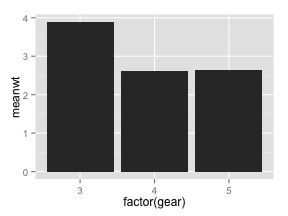Another option for quick graphing is to use the built-in stat_summary() layer. Instead of summarizing data, we use the original dataset and indicate that the x-axis is gear and the y-axis is just weight. However, we use stat_summary() to calculate the mean of the y for each x with the following command:

ggplot(mtc,aes(x=factor(gear), y=wt)) + stat_summary(fun.y=mean, geom="bar")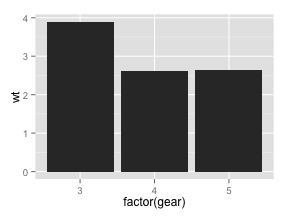There are reasons why we would want to use the first or second method. For the first, summarizing our data the way we want it gives us validity that we are sure that we are doing what we want to be doing and gives us more flexibility in case we want to use that summarized data in a later portion of our analysis (like in a table). Using the stat_summary() layer is faster and less code to write.

For now, we continue with the second method, but later on we'll come back to the summarizing method.

>> Horizontal bars, colors, width of bars

We can make these plots look more presentable with a variety of options. First, we rotate the bars so they are horizontal. Second, we change the colors of the bars. Finally, we change the width of the bars.

#1. horizontal bars
p1<-ggplot(mtc,aes(x=factor(gear),y=wt)) + stat_summary(fun.y=mean,geom="bar") +
coord_flip()

#2. change colors of bars
p2<-ggplot(mtc,aes(x=factor(gear),y=wt,fill=factor(gear))) +  stat_summary(fun.y=mean,geom="bar") +
scale_fill_manual(values=c("purple", "blue", "darkgreen"))

#3. change width of bars
p3<-ggplot(mtc,aes(x=factor(gear),y=wt)) +  stat_summary(fun.y=mean,geom="bar", aes(width=0.5))

grid.arrange(p1, p2, p3, nrow=1)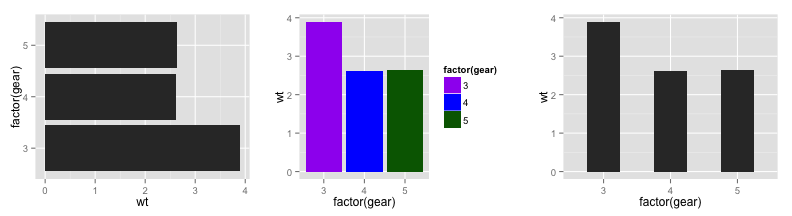For the colors, I color the bars by the gear variable so it's a different color for each bar, and then indicate manually the colors I want. You could color them all the same way using fill=“blue” for example, or you can keep the default colors when you fill by gear by leaving off scale_fill_manual altogether.

You can also use scale_fill_brewer() to fill the bars with a scale of one color (default is blue). This R cookbook site is particularly useful for understanding how to get the exact colors you want:http://www.cookbook-r.com/Graphs/Colors_(ggplot2)/

Note that if you are summarizing the data yourself, you change the width this way (graphs not shown since they look the same):

ggplot(summary.mtc, aes(x = factor(gear), y = meanwt)) + geom_bar(stat = "identity", width=0.2)

>> Split and color by another variable

Now, let's make the graph more complicated by adding a third variable. We can do this in three ways: bars next to each other, bars stacked, or using 'faceting' which is making multiple graphs at once. We would like to know the mean weight by both gear and engine type (vs). Stacking is a particularly bad idea in this example, but I'll show it for completeness.

#1. next to each other
p1<-ggplot(mtc,aes(x=factor(gear),y=wt,fill=factor(vs)), color=factor(vs)) +
stat_summary(fun.y=mean,position=position_dodge(),geom="bar")

#2. stacked
p2<-ggplot(mtc,aes(x=factor(gear),y=wt,fill=factor(vs)), color=factor(vs)) +
stat_summary(fun.y=mean,position="stack",geom="bar")

#3. with facets
p3<-ggplot(mtc,aes(x=factor(gear),y=wt,fill=factor(vs)), color=factor(vs)) +
stat_summary(fun.y=mean, geom="bar") +
facet_wrap(~vs)

grid.arrange(p1, p2, p3, nrow=1)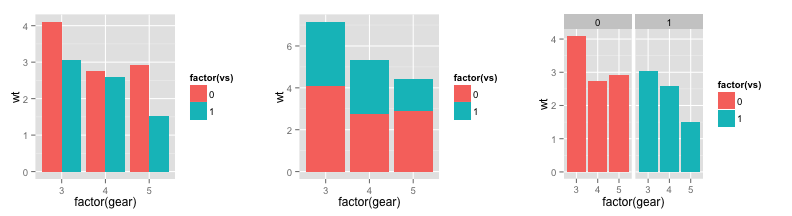You can also indicate the width of the spread between the bars in the first plot using position=position_dodge(width=.5) and play around with the width number.

You can change the order of the stacking by re-ordering the levels of the fill variable. Here is a prior blog post I had about how to reorder factors.

mtc\$vs2<-factor(mtc\$vs, levels = c(1,0))

ggplot(mtc,aes(x=factor(gear),y=wt,fill=factor(vs2)), color=factor(vs2)) +
stat_summary(fun.y=mean,position="stack",geom="bar")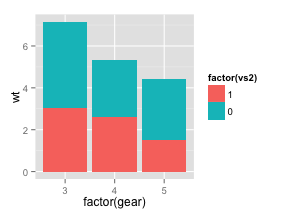Note that if you are using summarized data, just indicate the position in the geom_bar() statement.

Faceting is a really nice feature in ggplot2 and deserves more space on this blog, but for now more information on how faceting works can be found here: http://www.cookbook-r.com/Graphs/Facets_(ggplot2)/

>> Add text to the bars, label axes, and label legend

Next, I would like to add the value in text to the top of each bar. This is a case in which you definitely want to summarize the data first - it is much easier and cleaner that way. I use the aggregate() function to summarize the data by both gear and type of engine.

ag.mtc<-aggregate(mtc\$wt, by=list(mtc\$gear,mtc\$vs), FUN=mean)
colnames(ag.mtc)<-c("gear","vs","meanwt")
ag.mtc
##   gear vs meanwt
## 1    3  0  4.104
## 2    4  0  2.748
## 3    5  0  2.913
## 4    3  1  3.047
## 5    4  1  2.591
## 6    5  1  1.513

Now, I use the geom_bar() layer as in the first example, and the geom_text() layer to add the text. In order to move the text to the top of each bar, I use the position_dodge and vjust options to move the text around.

The first plot shows the basic output, but we see that the first number is cutoff by the top of the y-axis and we need to round the text. We can fix it by adjusting the range of the y-axis exactly how we did in a scatterplot, by adding a scale_y_continuous layer to the plot. I also change the x-axis label using scale_x_discrete, change the text to be black so it's readable, and label the legend. Notice here, it is the scale_fill_discrete layer.

Go back to the cheatsheet for scatterplots if you want to go over how to customize axes and legends.

#1. basic
g1<-ggplot(ag.mtc, aes(x = factor(gear), y = meanwt, fill=factor(vs),color=factor(vs))) +
geom_bar(stat = "identity", position=position_dodge()) +
geom_text(aes(y=meanwt, ymax=meanwt, label=meanwt),position= position_dodge(width=0.9), vjust=-.5)

#2. fixing the yaxis problem, changing the color of text, legend labels, and rounding to 2 decimals
g2<-ggplot(ag.mtc, aes(x = factor(gear), y = meanwt, fill=factor(vs))) +
geom_bar(stat = "identity", position=position_dodge()) +
geom_text(aes(y=meanwt, ymax=meanwt, label=round(meanwt,2)), position= position_dodge(width=0.9), vjust=-.5, color="black") +
scale_y_continuous("Mean Weight",limits=c(0,4.5),breaks=seq(0, 4.5, .5)) +
scale_x_discrete("Number of Gears") +
scale_fill_discrete(name ="Engine", labels=c("V-engine", "Straight engine"))

grid.arrange(g1, g2, nrow=1)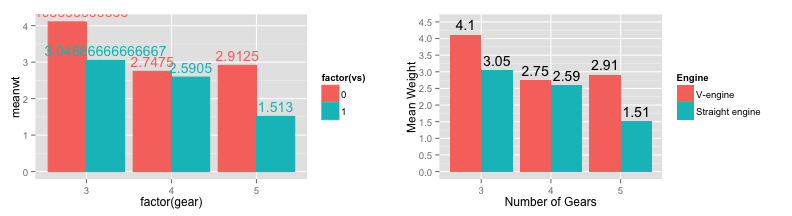>> Add error bars or best fit line

Again there are two ways to do this, but I prefer summarizing the data first and then adding in error bars. I use tapply to get the mean and SD of the weight by gear, then I add a geom_bar layer and a geom_errorbar layer, where I indicate the range of the error bar using ymin and ymax in the aes() statement.

summary.mtc2 <- data.frame(
gear=levels(as.factor(mtc\$gear)),
meanwt=tapply(mtc\$wt, mtc\$gear, mean),
sd=tapply(mtc\$wt, mtc\$gear, sd))
summary.mtc2
##   gear meanwt     sd
## 3    3  3.893 0.8330
## 4    4  2.617 0.6327
## 5    5  2.633 0.8189
ggplot(summary.mtc2, aes(x = factor(gear), y = meanwt)) +
geom_bar(stat = "identity", position="dodge", fill="lightblue") +
geom_errorbar(aes(ymin=meanwt-sd, ymax=meanwt+sd), width=.3, color="darkblue")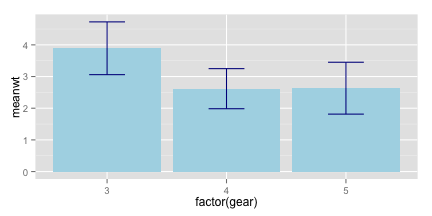And if you were really cool and wanted to add a linear fit to the barplot, you can do it in two ways. You can evaluate the linear model yourself, and then use geom_abline() with an intercept and slope indicated. Or you can take advantage of the stat_summary() layer to summarize the data and the geom_smooth() layer to add a linear model instantly.

#summarize data
summary.mtc3 <- data.frame(
hp=levels(as.factor(mtc\$hp)),
meanmpg=tapply(mtc\$mpg, mtc\$hp, mean))

#run a model
l<-summary(lm(meanmpg~as.numeric(hp), data=summary.mtc3))

#manually entering the intercept and slope
f1<-ggplot(summary.mtc3, aes(x = factor(hp), y = meanmpg)) +
geom_bar(stat = "identity",  fill="darkblue")+
geom_abline(aes(intercept=l\$coef[1,1], slope=l\$coef[2,1]), color="red", size=1.5)

#using stat_smooth to fit the line for you
f2<-ggplot(summary.mtc3, aes(x = factor(hp), y = meanmpg)) +
geom_bar(stat = "identity",  fill="darkblue")+
stat_smooth(aes(group=1),method="lm", se=FALSE, color="orange", size=1.5)

grid.arrange(f1, f2, nrow=1)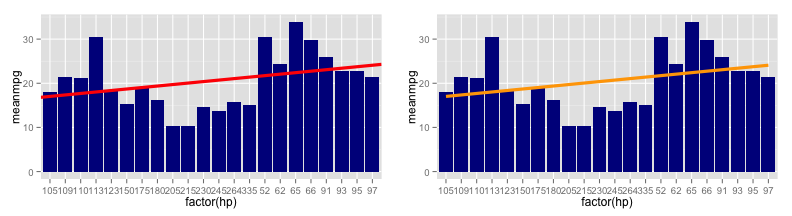And as before, check out The R cookbook and the ggplot2 documentation for more help on getting the bargraph of your dreams.

1.